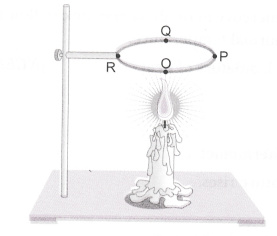# A circular metal loop is heated at point O as shown in the figure below

A circular metal loop is heated at point O as shown in the figure below.1. In which direction would heat flow in the loop?
2. In which order the pins at points P, Q and R fixed with the help of wax fall if points O, P, Q and R are equidistant from each other?